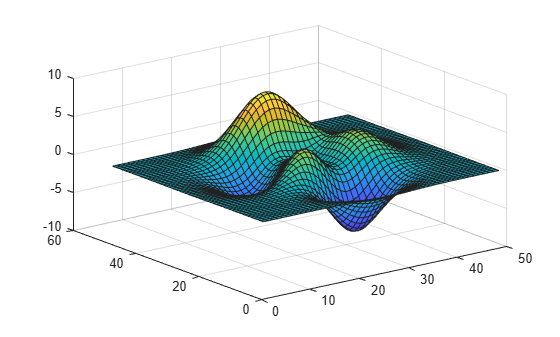Documentation

# brighten

Brighten or darken colormap

## Syntax

``brighten(beta)``
``brighten(map,beta)``
``newmap = brighten(___)``
``brighten(f,beta)``

## Description

example

````brighten(beta)` shifts the intensities of all colors in the current colormap in the same direction. The colors brighten when `beta` is between `0` and `1`, and they darken when `beta` is between `-1` and `0`. The magnitude of the change is proportional to the magnitude of `beta`. Use this syntax to adjust colors for all graphics objects in the current figure that use a colormap.```

example

````brighten(map,beta)` shifts the intensities of the colormap specified as `map`.```
````newmap = brighten(___)` returns the adjusted colormap for any of the input argument combinations in the previous syntaxes. When you specify the output argument, the current figure is not affected.```
````brighten(f,beta)` shifts the intensities of the colormap assigned to figure `f`. The colors of other graphics objects are affected, such as the axes, axes labels, and ticks.```

## Examples

collapse all

Create a surface plot with the default colormap.

`surf(peaks);`Brighten the colormap for current figure.

`brighten(.8);`Display two surface plots using the `subplot` function and the `summer` colormap.

```ax1 = subplot(1,2,1); surf(ax1,peaks); axis tight ax2 = subplot(1,2,2); surf(ax2,peaks); axis tight colormap(summer)```Darken the `summer` colormap and apply it to the second surface.

```newmap = brighten(summer,-.7); colormap(ax2,newmap)```## Input Arguments

collapse all

Brightness adjustment parameter, specified as a numeric scalar value. The `brighten` function raises each value in the colormap to the power of γ, which is defined as:

`$\gamma =\left\{\begin{array}{cc}1-\beta ,& \beta >0\\ \frac{1}{1+\beta },& \beta \le 0\end{array}$`

Data Types: `single` | `double`

Figure to adjust, specified as a `Figure` object. When you specify this argument, the colors of other graphics objects within the figure are affected in addition to the objects that use the colormap. For example, color of the axes, axes labels, and ticks changes.

Colormap to adjust, specified as a three-column matrix of RGB triplets. An RGB triplet is a three-element row vector whose elements specify the intensities of the red, green, and blue components of a color. The intensities must be in the range [0, 1]. For example, here is a colormap that contains five colors:

```map = [0.2 0.1 0.5 0.1 0.5 0.8 0.2 0.7 0.6 0.8 0.7 0.3 0.9 1 0];```

This table lists the RGB triplet values for common colors.

ColorRGB Triplet
yellow`[1 1 0]`
magenta`[1 0 1]`
cyan`[0 1 1]`
red`[1 0 0]`
green`[0 1 0]`
blue`[0 0 1]`
white`[1 1 1]`
black`[0 0 0]`

Alternatively, you can create the matrix by calling one of the predefined colormap functions. Call the function as an input argument to the `brighten` function. For example, this command brightens the `parula` colormap.

`brighten(parula,.8)`

Data Types: `double` | `single`

## Output Arguments

collapse all

Adjusted colormap, returned as a three-column matrix of RGB triplets.

Download ebook